• 软件库：scipy.optimize, numpy, CVXPY,Gekko 软件：octave 5.1，matlab 本文将介绍三种计算非线性约束优化的方法： （1）scipy.optimize.minimize （2）cvxpy （3）Octave 5.1 sqp函数 （4）matlab ga函数 博主其他...
软件库：scipy.optimize, numpy, CVXPY,Gekko
软件：octave 5.1，matlab
本文将介绍三种计算非线性约束优化的方法：
（1）scipy.optimize.minimize
（2）cvxpy
（3）Octave 5.1 sqp函数
（4）matlab ga函数
博主其他相关博文：
CVXPY/Scipy/Octave线性规划问题求解

2020-08-13 更新
使用cvxpy库的时候，对于有些优化问题需要注意转换一下形式，例如下面二次规划问题(https://www.inverseproblem.co.nz/OPTI/index.php/Probs/QP)：需要先将优化问题转成二次规划的一般形式：
$\text{min} f(\bold{x})=\frac{1}{2} \bold{x}'\bold{H}\bold{x}+\bold{f}'\bold{x}$
其中$\bold{x}$是列向量，可以算出$\bold{H}=\begin{bmatrix} 1 & -1 \\ -1 & 2\end{bmatrix}$，$\bold{f}=\begin{bmatrix} -2 \\ -6 \end{bmatrix}$，相应的cvxpy计算程序如下（参考官方例子https://www.cvxpy.org/examples/basic/quadratic_program.html）：
import numpy as np
import cvxpy as cvx
x = cvx.Variable(2)
H = np.array([[1,-1],
[-1,2]])
f = np.array([-2,-6])
A = np.array([[1,1],
[-1,2],
[2,1]])
b = np.array([2,2,3])

# obj = cvx.Minimize(1/2*x**2+x**2-x*x-2*x-6*x)
cons = [A@x<=b, x>=0]

prob = cvx.Problem(obj, cons)
prob.solve()

print(prob.status, prob.value, x.value)

在上面例子中，如果采用obj = cvx.Minimize(1/2*x**2+x**2-x*x-2*x-6*x)计算会提示求解错误，需要处理为obj = cvx.Minimize((1/2)*cvx.quad_form(x,H)+f.T@x)形式，具体为什么前面的形式无法求解，笔者也还需进一步学习。

2019-10-19补充Gekko库非线性约束优化
使用Gekko优化计算库求解博文中的一个非线性约束优化例子（无法用cvxpy求解，报错提示优化问题不遵循DCP规则），程序代码如下：
from gekko import GEKKO
import time

start = time.time_ns()
m = GEKKO() # Initialize gekko
# Use IPOPT solver (default)
m.options.SOLVER = 3
# Change to parallel linear solver
# m.solver_options = ['linear_solver ma97']
# Initialize variables
x1 = m.Var(value=1,lb=0,ub=5)
x2 = m.Var(value=2,lb=0,ub=5)

# Equations
m.Equation(x1**2+x2**2<=6)
m.Equation(x1*x2==2)

m.Obj(x1**3-x2**2+x1*x2+2*x1**2) # Objective

m.options.IMODE = 3 # Steady state optimization

m.solve(disp=False) # Solve
print('Results')
print('x1: ' + str(x1.value))
print('x2: ' + str(x2.value))
end = time.time_ns()

print('Objective: ' + str(m.options.objfcnval))
print('Time used: {:.2f} s'.format((end-start)*1e-9))
'''
Results
x1: [0.87403204792]
x2: [2.2882456138]
Objective: -1.040502879
Time used: 24.37 s
'''

相比于scipy.optimize.minimize和octave，使用gekko求解该问题耗时很久，估计是解法器没有选择到最优，因为刚接触gekko，该问题后面再研究。
Gekko默认使用公共服务器资源进行计算，网络传输会影响程序执行速度，在初始化Gekko时可设置为本地计算求解，只要设置m = Gekko(remote=False)即可，执行结果为：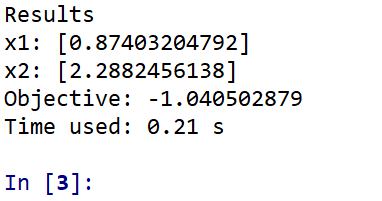MATLAB ga函数
2019-05-10更新
matlab有自带的遗传算法函数ga，相关语法如下图：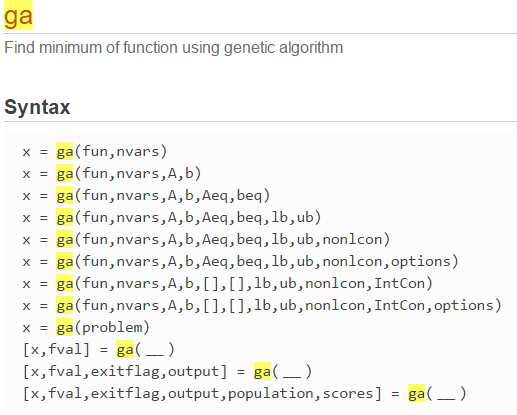octave有个ga工具箱，同样提供了ga函数，程序和前面的类似，更改一下函数定义的位置即可。
对于下面octave中求解Himmelblau函数：
$f(x,y)=(x^{2} +y-11)^{2}+(x+y^2-7)^2$的最优解，在matlab中程序如下：
%% MATLAB ga求解最优化问题

lb=[-5,-5];
ub=[5,5];

%% initial guess
x0 = [-3,-2];
%% MATLAB ga函数，详细信息请查看帮助文档
x  = ga (@phi,2,[],[],[],[],lb,ub)

%% object function，matlab中函数定义要放电一个脚本的最后面，这和octave脚本函数位置要求不同
function obj = phi (x)
obj = (x(1)^2+x(2)-11)^2+(x(1)+x(2)^2-7)^2;
end


结果为:
x = 1×2
3.0000    2.0000


从结构可以看出像ga这种进化算法只得到了一个最优解。因为matlab中的ga函数还可以处理线性约束和非线性约束问题，那么我们继续求解下文中的两个例子。
Example1
注意A，b系数矩阵要转换为Ax<=b的格式
% MATLAB ga求解最优化问题，注意A，b系数矩阵要转换为Ax<=b的格式
A = [-1,2;1,2;1,-2];
b = [2;6;2];
lb=[0,0];
ub=[];
option = optimoptions('ga','PlotFcn',@gaplotbestf)
%% MATLAB ga函数

x  = ga (@phi,2,A,b,[],[],lb,ub,[],option)

%% object function

function obj = phi (x)
obj = (x(1)-1)^2+(x(2)-2.5)^2;
end


结果为：
x = 1×2
1.4224    1.7117


可见其结果离真实解还有一定偏差，为此我们可以控制ga的option来提供精度，将option修改为如下：
option = optimoptions('ga','ConstraintTolerance',1e-6,'PlotFcn',@gaplotbestf)

运行结果为：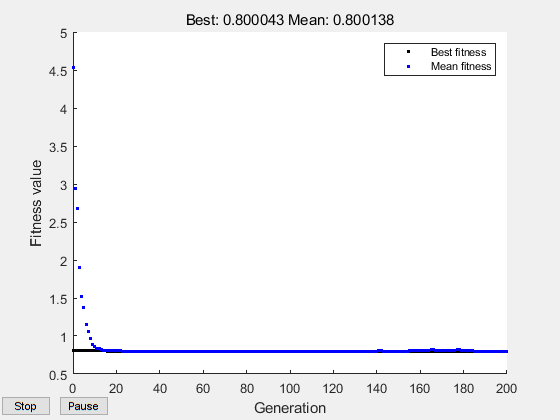x = 1×2
1.3941    1.6970


Example 2
option = optimoptions('ga','ConstraintTolerance',1e-6,'PlotFcn',@gaplotbestf)
%% MATLAB ga函数
x  = ga (@phi,2,[],[],[],[],[],[],@cons,option)

%% object function
function obj = phi (x)
obj = x(1)^3-x(2)^3+x(1)*x(2)+2*x(1)^2;
end

%% nonlinear constraints，非线性约束条件
function [c,ceq] = cons(x)
c = x(1)^2+x(2)^2-6; % Compute nonlinear inequalities at x，不等式约束，c(x)<=0
ceq = x(1)*x(2)-2;       % Compute nonlinear equalities at x，等式约束，ceq(x)=0
end

结果如下：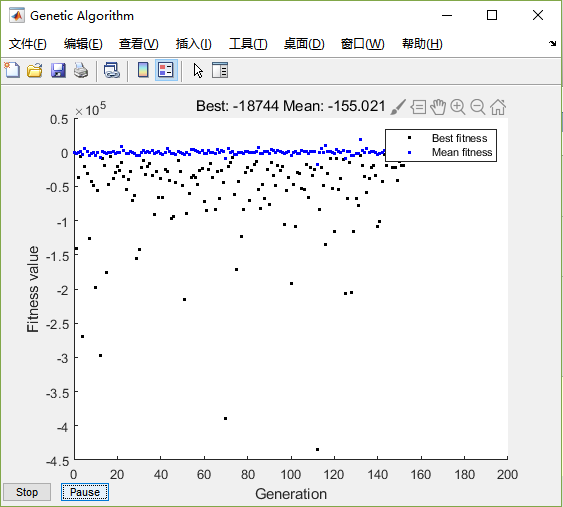Optimization terminated: stall generations limit exceeded
but constraints are not satisfied.
x =
1.0255    1.9512

可见计算结果并不满足约束条件，也和真实解（x1 = 0.8740320488968545
x2 = 2.2882456112715115）相差比较大。
ga作为一种随机性的进化算法，ga本身的参数（如crossover、mutation等）都会对结果造成很大影响，这里就不继续探讨了。
scipy.optimize.minimize
相关函数：
scipy.optimize.minimize(fun, x0, args=(), method=None, jac=None, hess=None, hessp=None, bounds=None, constraints=(), tol=None, callback=None, options=None)
func：求解的目标函数，Python callable function
x0：给定的初始值
args：目标函数中的参数
method：求解算法
bounds：边界约束
constraints：条件约束
其他详细内容请查看官方帮助文档。
这部分内容为scipy官方文档翻译过来，但官方文档对带约束的最优化问题介绍的比较少，其引用了一本书籍的内容，下面记录之。
（1）根据文档所知，参考书籍为Nocedal, J, and S J Wright. 2006. Numerical Optimization. Springer New York.
使用例子来自example16.3(p462)，文档有误，问题描述如下：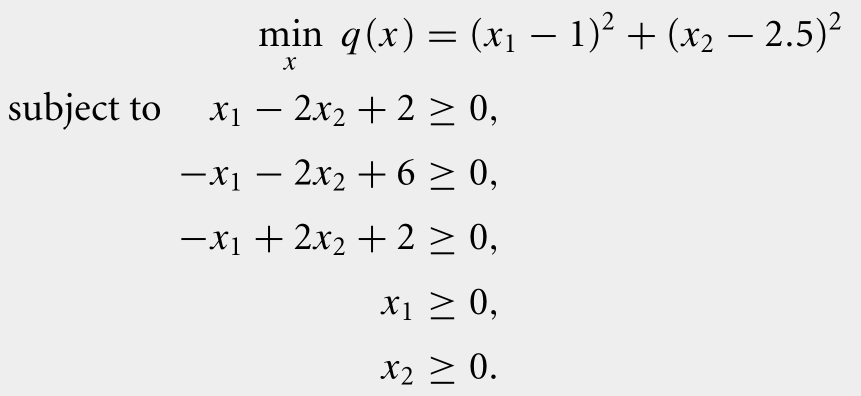代码如下：
import numpy as np
from scipy.optimize import minimize

# 目标函数
def objective(x):
return (x - 1)**2 + (x - 2.5)**2

# 约束条件
def constraint1(x):
return x - 2 * x + 2  #不等约束

def constraint2(x):

return -x - 2 * x + 6 #不等约束

def constraint3(x):

return -x + 2 * x + 2 #不等约束

# 初始猜想
n = 2
x0 = np.zeros(n)
x0 = 2
x0 = 0

# show initial objective
print('Initial SSE Objective: ' + str(objective(x0)))

# 边界约束
b = (0.0,None)
bnds = (b, b) # 注意是两个变量都要有边界约束

con1 = {'type': 'ineq', 'fun': constraint1}
con2 = {'type': 'ineq', 'fun': constraint2}
con3 = {'type': 'ineq', 'fun': constraint3}
cons = ([con1,con2,con3]) # 3个约束条件

# 优化计算
solution = minimize(objective,x0,method='SLSQP',\
bounds=bnds,constraints=cons)
x = solution.x

# show final objective
print('Final SSE Objective: ' + str(objective(x)))

# print solution
print('Solution')
print('x1 = ' + str(x))
print('x2 = ' + str(x))


结果如下：
Initial SSE Objective: 7.25
Final SSE Objective: 0.8000000011920985
Solution
x1 = 1.4000000033834283
x2 = 1.7000000009466527

就是当x1=1.4,x2=1.7时在约束条件下目标函数取得最小值。
再举一个例子加深印象，参照https://jingyan.baidu.com/album/6c67b1d69508b52787bb1edf.html?picindex=2，求解下面优化问题：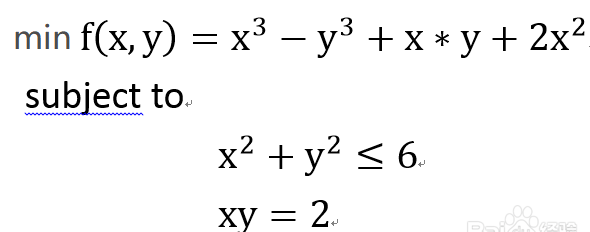使用python代码如下：
import numpy as np
from scipy.optimize import minimize

def objective(x):
return x**3-x**3+x*x+2*x**2

def constraint1(x):
return -x**2 - x**2 + 6.0

def constraint2(x):

return 2.0-x*x

# initial guesses
n = 2
x0 = np.zeros(n)
x0 = 1
x0 = 2

# show initial objective
print('Initial SSE Objective: ' + str(objective(x0)))

# optimize
b = (0.0,3.0)
bnds = (b, b)
con1 = {'type': 'ineq', 'fun': constraint1}
con2 = {'type': 'eq', 'fun': constraint2}
cons = ([con1,con2])
solution = minimize(objective,x0,method='SLSQP',\
bounds=bnds,constraints=cons)
x = solution.x

# show final objective
print('Final SSE Objective: ' + str(objective(x)))

# print solution
print('Solution')
print('x1 = ' + str(x))
print('x2 = ' + str(x))


结果如下：
Initial SSE Objective: -3.0
Final SSE Objective: -7.785844454002188
Solution
x1 = 0.8740320488968545
x2 = 2.2882456112715115

和原作者在matlab求解结果一致
特别注意： 使用约束的时候，要转化为形如ax+by>=c的形式，像前面第二个例子中的不等约束为$x^2+y^2<=6$，则要转化为$-x^2-y^2+6>=0$的形式，然后再编写约束函数的代码：
def constraint1(x):
return -x**2 - x**2 + 6.0

接着定义约束类型：
con1 = {'type': 'ineq', 'fun': constraint1}

cvxpy
使用cvxpy求解前面第一个问题，起程序如下：
import cvxpy as cp
import numpy as np

'''
minimize f(x)=(x-1)^2+(y-2.5)^2
s.t.
x-2y+2>=0
-x-2y+6>=0
-x+2y+2>=0
x>=0
y>=0
'''
A = np.array([[1, -2],
[-1, -2],
[-1, 2]])

b = np.array([-2, -6, -2])

x = cp.Variable(2)
prob=cp.Problem(cp.Minimize( (x-1)**2 + (x-2.5)**2),
[A@x>=b,x>=0,x>=0])
prob.solve()

# show final objective
print('Final SSE Objective: ' + str(prob.value))

# print solution
print('Solution of x and y: ' + str(x.value))


运行结果为：
Final SSE Objective: 0.8000000000000005
Solution of x and y: [1.4 1.7]


对于第二个例子笔者用cvxpy暂时解不出来，程序报错：
cvxpy.error.DCPError: Problem does not follow DCP rules. Specifically:
The objective is not DCP. Its following subexpressions are not:

因为求解不成功就不放出程序了，以后研究透了再更新。

Octave5.1
相关函数：
[…] = sqp (x0, phi, g, h, lb, ub, maxiter, tol)

该函数的官方文档介绍如下：
Minimize an objective function using sequential quadratic programming (SQP).
Solve the nonlinear program
min phi (x)
x
subject to
g(x)  = 0
h(x) >= 0
lb <= x <= ub
using a sequential quadratic programming method.

参数说明（重点）
（1）x0:初始猜想值，向量形式，如果是n个变量优化问题，x0也为n维向量；
（2）phi:目标函数，可以使用函数句柄
（3）g:等式约束条件，如果没有约束用空矩阵[]代替
（4）h：不等式约束条件，如果没有约束用空矩阵[]代替
（5）lb：变量左边界约束，同样注意和变量个数有关
（6）ub：变量右边界约束，和变量个数有关
先举个简单的例子，目标函数为Himmelblau函数，关于了解更多优化计算中测试算法使用的函数，可以参考网站http://infinity77.net/global_optimization/index.html下面的Test Function部分内容，也可在维基百科中搜索，这里就不过多介绍了。
Himmelblau函数：
$f(x,y)=(x^{2} +y-11)^{2}+(x+y^2-7)^2$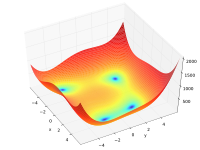使用octave求解代码如下：
%%OCTAVE脚本里面有函数时，需要先有其他语句开始，不然就会当做是函数文件
1;
%% object function
function obj = phi (x)
obj = (x(1)^2+x(2)-11)^2+(x(1)+x(2)^2-7)^2;
endfunction
lb=[-5,-5];
ub=[-5,5];

%% initial guess
x0 = [-3,-2];
%% 没有约束条件，用[]代替
[x, obj, info, iter, nf, lambda] = sqp (x0, @phi, [],[],lb,ub)

结果如下：
>> nonlinear_prob

x =

-3.7793
-3.2832

obj =    1.5554e-14
info =  104
iter =  8
nf =  17
lambda =

0
0
0
0

可见在初始值（-3，-2）情况下找到了最优解（-3.7793，-3.2832）
下面求解前面带约束的例子：
1;
%% equality constraints
function r = g (x)
r = [ x(1)*x(2)-2];
endfunction

function r = h (x)
r = [ 6-x(1)^2-x(2)^2];
endfunction

%% object function
function obj = phi (x)
obj = x(1)^3-x(2)^3+x(1)*x(2)+2*x(1)^2;
endfunction
lb=[0,0];
ub=[3,3];

%% initial guess
x0 = [1,1];

[x, obj, info, iter, nf, lambda] = sqp (x0, @phi, @g, @h, lb, ub)


结果如下：

x =

0.87403
2.28825

obj = -7.7858
info =  104
iter =  6
nf =  8
lambda =

7.03150
4.58428
0.00000
0.00000
0.00000
0.00000

计算得出的x解和scipy.optimize求出的一致，这点以后有时间再验证一下。
————
2019-04-17补充
优路径最短问题可以归结为最优化问题，即求解一个点到其他点距离的最小值。
minimize
$minimize f(x,y)=\sum((x-datax_i)^2+(y-datay_i)^2)$
这里为了方便计算，使用了距离的平方，没有开根号计算。
根据前面的目标函数，我们写成符合scipy.optimzie.minimize的函数输入格式，最后完整的程序如下：
import numpy as np
from scipy.optimize import minimize
import matplotlib.pyplot as plt

datax = 10*np.random.randn(15)
datay = 10*np.random.rand(15)

def f(x):
return sum((x-datax[i])**2+(x-datay[i])**2 for i in range(len(datax)))

# 初值
x0 = [1,1]
# 计算结果
opt = minimize(f,x0=x0)
print(opt)

plt.figure()
plt.scatter(datax,datay,label='Original points')
plt.scatter(opt.x, opt.x, s=240, marker='h',label='Center point')
plt.legend()
# plt.show()
for i in range(len(datax)):
plt.plot([opt.x,datax[i]],[opt.x,datay[i]])
plt.show()

结果如下：
      fun: 762.1598697487602
hess_inv: array([[ 3.33359580e-02, -8.50862577e-06],
[-8.50862577e-06,  3.33328322e-02]])
jac: array([ 0.00000000e+00, -7.62939453e-06])
message: 'Optimization terminated successfully.'
nfev: 28
nit: 5
njev: 7
status: 0
success: True
x: array([0.89233268, 5.09306968])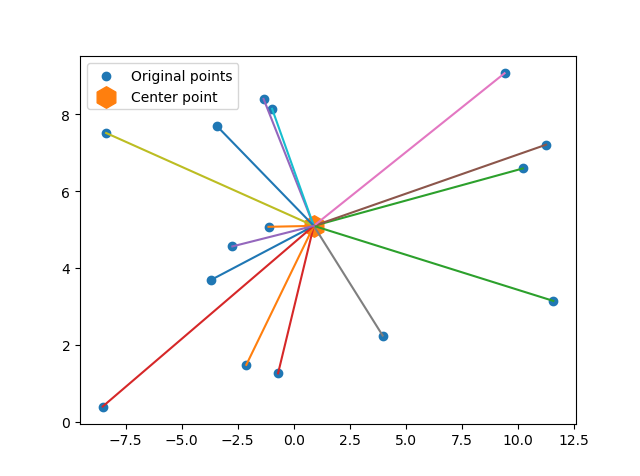CVXPY
import cvxpy as cp
import numpy as np
import matplotlib.pyplot as plt
datax = [2.5,6.7,3,9.3,9.2,9,4,7.2,0.3,2.8,10.3,2.4,5.7,8.1,3.5,6.89,1.34]
datay = [5.7,6.2,5.0,7.9,2.7,9.3,8,2,9,4,6.8,1.9,3.8,7.2,5.71,4.88,2.3]

def f(x):
return sum((x-datax[i])**2+(x-datay[i])**2 for i in range(len(datax)))

x = cp.Variable(2)
prob=cp.Problem(cp.Minimize(sum((x-datax[i])**2+(x-datay[i])**2 for i in range(len(datax)))))
prob.solve()

# show final objective
print('Final SSE Objective: ' + str(prob.value))

# print solution
print('Solution of x and y: ' + str(x.value))

plt.figure()
plt.scatter(datax,datay,label='Original points')
plt.scatter(x.value, x.value, s=240, marker='h',label='Center point')
plt.legend()
# plt.show()
for i in range(len(datax)):
plt.plot([x.value,datax[i]],[x.value,datay[i]])
plt.show()


对于求解线性规划问题，请参考文首给出的链接。
参考链接：
https://docs.scipy.org/doc/scipy/reference/generated/scipy.optimize.minimize.html#rdd2e1855725e-5
https://apmonitor.com/pdc/index.php/Main/NonlinearProgramming
https://jingyan.baidu.com/article/6c67b1d69508b52787bb1edf.html


展开全文minimize
• Nlopt在vs2013、2015中配置 ... 【注意：由于后来在vs环境下是用win32平台编译的，所以要下载32bit版本；如果你在vs环境下是用X64平台编译，则下载64bit版本；一定要注意这里不是根据电脑的版本或安装vs的版本来选择，...
Nlopt在vs2013、2015中配置

在http://ab-initio.mit.edu/wiki/index.php/NLopt_on_Windows下载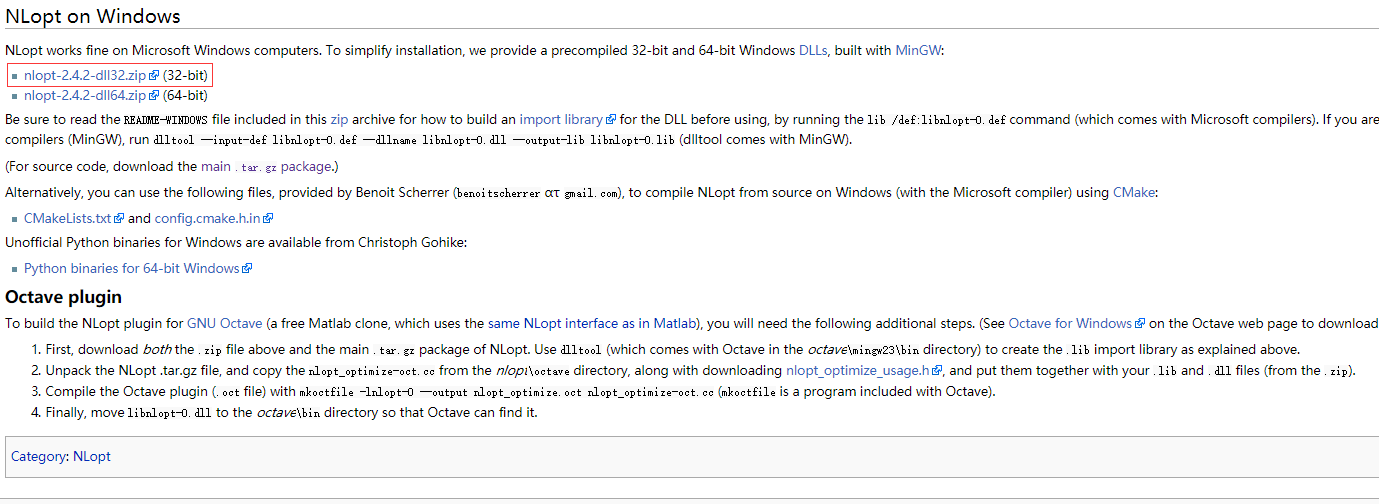【注意：由于后来在vs环境下是用win32平台编译的，所以要下载32bit版本；如果你在vs环境下是用X64平台编译，则下载64bit版本；一定要注意这里不是根据电脑的版本或安装vs的版本来选择，否则后面调试会出现如下问题】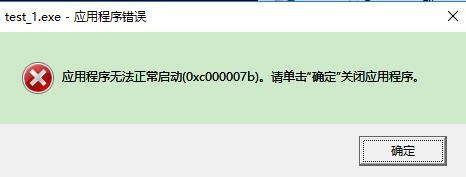在开始菜单里，vs编译器工具
【针对vs2015】因为是32bit版本，所以选择X86版本的，但本人也是编程小白，为了保险起见，就选择了如下：（vs2013类似）输入：cd F:\nonlinearoptimal\nlopt-2.4.2-dll32（你所下载的dll32文件夹的路径）
然后 F: (小白作者担心是否打开了文件夹，就输入了文件夹所在的盘符，进入了该文件夹)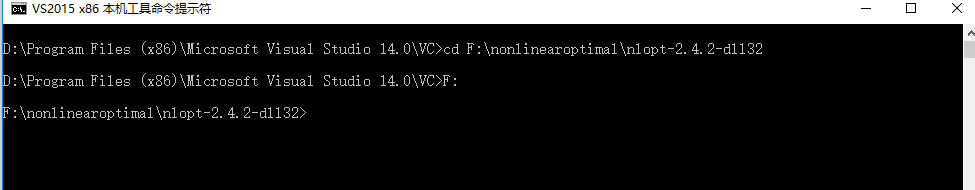输入：lib /def:libnlopt-0.def
运行完成之后，就会在该dll32文件夹内生成.lib文件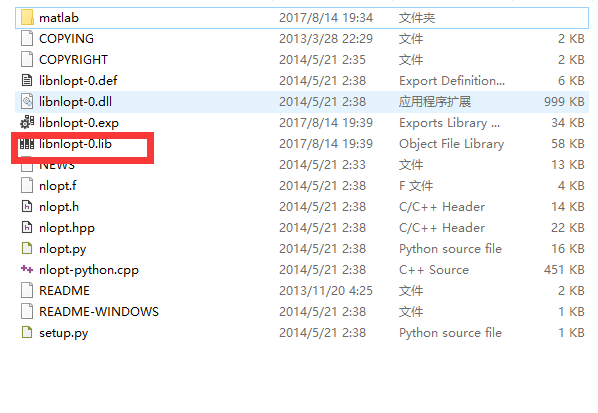打开vs2015，新建一个空项目，然后对其项目属性进行配置，方法如同【在vs中配置第三方库】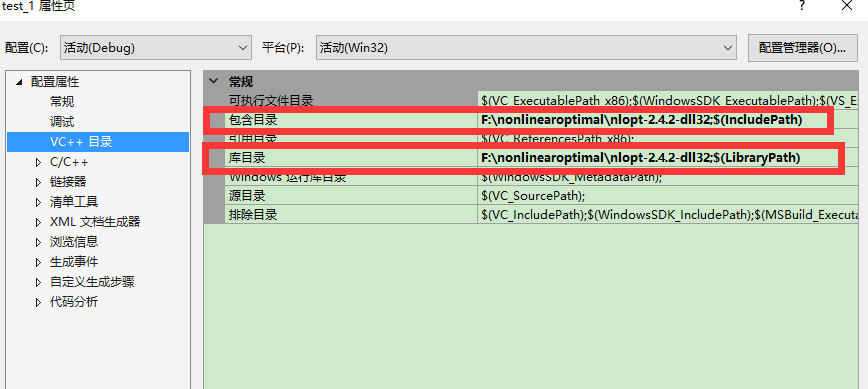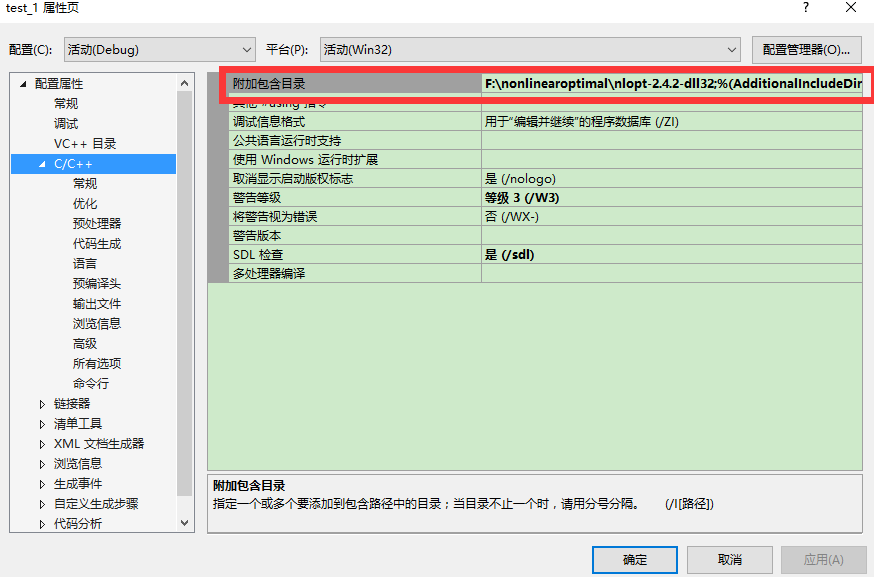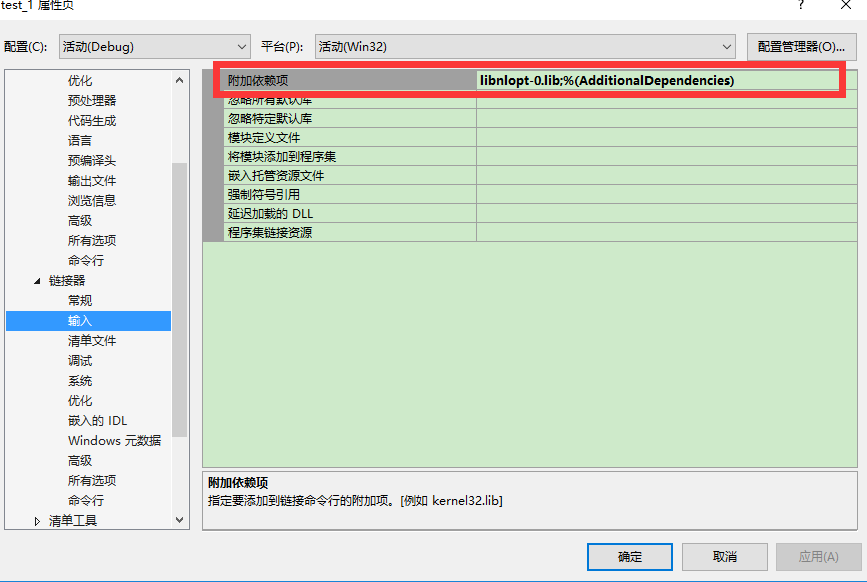要想调用该库时，将dll32文件下的头文件.h/.hpp也加入到该项目中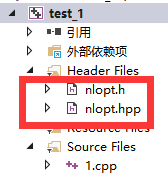最后将dll32文件下的.dll文件复制到你所安装的vs2013或vs2015的目录下，找到vc文件夹下的bin文件夹。【以vs2015为例，vs2013安装文件则是12.0】然后对示例程序进行编译即可成功。
作者小白参考了诸多教程，希望和大家共享经验教训哈哈哈

展开全文vs2015 vs2013
• 概述 在实际问题中不是所有的目标函数或者约束都是线性的，本节主要介绍对于非线性约束...非线性约束优化问题 基本形式表示为min f(x) s.t ci(x)=0,i∈Eci(x)≥0,i∈Imin\ f(x)\ \\ s.t\ \ c_i(x)=0, i \in
概述

在实际问题中不是所有的目标函数或者约束都是线性的，本节主要介绍对于非线性约束或者目标函数如何有效的求解。
1. 非线性约束问题概述
2. 求解非线性约束常用的思路
3. 总结

非线性约束最优化问题

基本形式表示为min f(x) s.t  ci(x)=0,i∈Eci(x)≥0,i∈I<!--//--><![CDATA[//><!--
min\ f(x)\ \\ s.t\ \ c_i(x)=0, i \in \mathcal E \\ c_i(x)\ge 0, i \in \mathcal I
//--><!]]>该类问题的最优解一阶和二阶条件都已介绍过。
后续会介绍几类常见的非线性约束最优化问题
2. 带惩罚的增强拉格朗日算法，主要思路将目标函数和约束整合到一起，通过求解一系列无约束问题从而逼近原始问题的解。例如对于等式约束问题，可以定义F(x)=f(x)+u2∑i∈Eci(x)2<!--//--><![CDATA[//><!--
F(x)=f(x)+\frac u2 \sum_{i \in \mathcal E}c_i(x)^2
//--><!]]>。或者F(x)=f(x)+u∑i∈E|ci(x)|<!--//--><![CDATA[//><!--
F(x)=f(x)+u \sum_{i \in \mathcal E}|c_i(x)|
//--><!]]>。对于增强拉格朗日方法，综合考虑原始目标和约束，例如L=f(x)+u2∑i∈Eci(x)2−∑i∈Eλici(x)<!--//--><![CDATA[//><!--
\mathcal L=f(x)+\frac u2 \sum_{i \in \mathcal E}c_i(x)^2 -\sum_{i \in \mathcal E}\lambda_ic_i(x)
//--><!]]>
min \frac 12 p^T \nabla^2\mathcal L(x_k,\lambda_k)p+\nabla f(x_k)^Tp\\s.t \ \nabla c_i(x_k)^Tp+c_i(x_k)=0, i \in \mathcal E\\\nabla c_i(x_k)^Tp+c_i(x_k)\ge 0, i \in \mathcal I
//--><!]]>相当于在某个步骤中对拉格朗日进行泰勒展开，计算最佳搜索方向。
4. 内点法，也成为障碍方法，将约束添加到目标函数中，从而通过求解无约束问题得到最优解。

常用求解思路

不等式约束处理

在有效集算法（Active Set Method）中，类似于单纯形算法，每一次求解仅仅考虑等式约束。主要思路如下
1. 定义某个工作集合W为要满足的等式约束
2. 求解满足等式约束集合W，对于原始问题的最优解，检查是否满足不等式约束。
3. 将某个不等式约束，变成等式约束加入到W集合中
4. 不断重复第二部步骤，直到找到最优解。

通过这类算法可以只处理等式约束就可以找到最优解，但是复杂度相对较大，例如对于n个不等式约束，就要重复2^n步。 问题即使遍历了所有不等式约束也有可能找不到最优解

变量消减方法

对于等式约束问题，可以将等式约束进行转换代入到目标函数中，问题是某些等式约束可能会带有隐式约束，如果不能处理，则直接导致原始问题不可解。

对于现行约束，例如Ax=b<!--//--><![CDATA[//><!--
Ax=b
//--><!]]>可以直接采用变量消减或者高斯消元法进行求解

价值函数(Merit Function)

在很多求解步骤中，要不断的平衡目标函数和约束函数，例如某个搜索步骤带来较大的目标函数减少，但是会带来更多的约束函数不满足，此时就要利用价值函数进行平衡。
价值函数就是在原始目标函数上添加对约束函数的惩罚项。

精确的价值函数，对于添加了惩罚项的ϕ(x;u)<!--//--><![CDATA[//><!--
\phi(x;u)
//--><!]]>，如果对于存在u∗<!--//--><![CDATA[//><!--
u^*
//--><!]]>，使得任何u>u∗<!--//--><![CDATA[//><!--
u>u^*
//--><!]]>情况下，原始问题的解都是修改后问题的解。

常见的价值函数 ϕ1(x;u)=f(x)+u∑i∈E|ci(x)|+u∑i∈I[ci(x)]−<!--//--><![CDATA[//><!--
\phi_1(x;u)=f(x)+u\sum_{i\in\mathcal E}|c_i(x)|+u\sum_{i\in \mathcal I}[c_i(x)]^-
//--><!]]>其中[x]−=max(0,−x)<!--//--><![CDATA[//><!--
[x]^-=max(0,-x)
//--><!]]>ϕ2(x;u)=f(x)+u||c(x)||2<!--//--><![CDATA[//><!--
\phi_2(x;u)=f(x)+u||c(x)||_2
//--><!]]>ϕF(x;u)=f(x)+λ(x)Tc(x)+12u∑i∈E[ci(x)]2<!--//--><![CDATA[//><!--
\phi_F(x;u)=f(x)+\lambda(x)^Tc(x)+\frac 12 u\sum_{i\in \mathcal E}[c_i(x)]^2
//--><!]]>Filter 方法

思路来源于多目标优化，即定义惩罚项h(x)=u∑i∈E|ci(x)|+u∑i∈I[ci(x)]−<!--//--><![CDATA[//><!--
h(x)=u\sum_{i\in\mathcal E}|c_i(x)|+u\sum_{i\in \mathcal I}[c_i(x)]^-
//--><!]]>
同时优化两个目标 min f(x)和min h(x)

Maratos 影响

Maratos Effect在很多问题中都会遇到，特别是使用价值函数的方法中，即要同时满足约束和最优化目标函数（每一步使得目标函数值下降），可能存在某个步骤不满足约束或者目标值不下降，但是沿着该步骤能够找到最优解。
对于任何使用价值函数的方法ϕ(x,u)=f(x)+uh(c((x))<!--//--><![CDATA[//><!--
\phi(x,u)=f(x)+uh(c((x))
//--><!]]>，如果h(0)=0，则一定会受到maratos影响。
改进方案有

使用一个不受影响的价值函数，例如h(x) != 0
添加一个二阶修正项，pk+p^k<!--//--><![CDATA[//><!--
p_k+\hat p_k
//--><!]]>
使用非单调策略，不是每一个接受的搜索方向都使得目标函数值下降，一定概率接受其他。例如WatchDog策略，对于某个搜索方向，尝试t=5-8次，如果不能找到大幅度下降的解，则返回原始步骤继续求解。

总结

该小结主要介绍了非线性约束最优化问题形式，和基本求解策略。
展开全文• ##fmincon函数非线性约束下的最优化问题 fmincon函数，既是求最小约束非线性多变量函数 该函数被用于求如下函数的最小值 语法如下: x = fmincon(fun,x0,A,b) x = fmincon(fun,x0,A,b,Aeq,beq) x = fmincon(fun,x0,...
fmincon函数非线性约束下的最优化问题
fmincon函数，既是求最小约束非线性多变量函数
该函数被用于求如下函数的最小值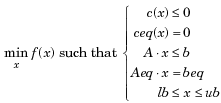语法如下:

x = fmincon(fun,x0,A,b)
x = fmincon(fun,x0,A,b,Aeq,beq)
x = fmincon(fun,x0,A,b,Aeq,beq,lb,ub)
x = fmincon(fun,x0,A,b,Aeq,beq,lb,ub,nonlcon)
x = fmincon(fun,x0,A,b,Aeq,beq,lb,ub,nonlcon,options)
x = fmincon(problem)
[x,fval] = fmincon(…)
[x,fval,exitflag] = fmincon(…)
[x,fval,exitflag,output] = fmincon(…)
[x,fval,exitflag,output,lambda] = fmincon(…)

对于fmincon函数，其exitflag参数中的数字：

1、一阶最优性条件满足容许范围
2、X的变化小于容许范围
3、目标函数的变化小于容许范围
4、重要搜索方向小于规定的容许范围并且约束违背小于options.TolCon
5、重要方向导数小于规定的容许范围并且约束违背小于options.TolCon
0、到达最大迭代次数或到达函数评价
-1、算法由输出函数终止
-2、无可行点

实例：求解下式的最小值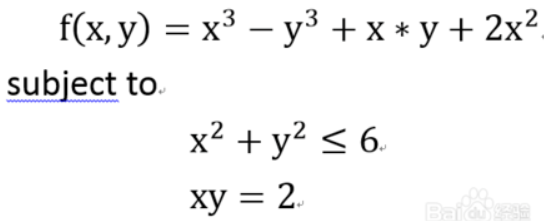%方式一：分成多个文件
function f=fminxy(t)
x=t(1);y=t(2);
f=x*x*x-y*y*y+2*x*x+x*y;
end
%%以上代码生成fminxy文件
function [c d]=fcontr(t)
x=t(1);y=t(2);
c=x*x+y*y-6;
d=x*y-2;
end
%%以上文件生成fcontr文件
>> [x,fval,exitflag]=fmincon('fminxy',[1 2],[],[],[],[],[],[],'fcontr')
%fmincon(	fun,	x0, A, b,Aeq,beq,lb,ub, nonlcon) A是初始值，fmincon的初始值x0可以任意取，只要保证为实数就行。
x =
0.8740     2.2882      %函数最小值时x,y的值
fval =
-7.7858                        %函数最小值
exitflag =
1                               %一阶最优性条件满足容许范围，既是结果正确

%方式二：整合成一个文件
function  x= findOpt(a,b)
x=fmincon(@(t) fminxy(t),[a b],[],[],[],[],[],[],@(t) fcontr(t))
end

function f=fminxy(t)
x=t(1);y=t(2);
f=x*x*x-y*y*y+2*x*x+x*y;
end

function [c d]=fcontr(t)
x=t(1);y=t(2);
c=x*x+y*y-6;
d=x*y-2;
end
%以上代码生成findOpt文件
>> x=findOpt(2,2)
x =
0.8740   2.2882


该实例皆为在MATLAB R2014a运行所得，参考https://jingyan.baidu.com/album/6c67b1d69508b52787bb1edf.html?picindex=4


展开全文• 借助老师的PPT对约束非线性优化问题的几何意义和对偶形式进行阐述。 一、几何意义 （1）等式约束 考虑只有等式约束h(x)的非线性优化问题，形式为： 可视化结果如下图所示，红色曲线为等式约束，环线为目标函数...
• 建立非线性规划的模型、无约束优化问题的建模与求解数学建模 matlab
• 这个matlab求解存在多个非线性不等式约束的多元约束优化问题方法真的很讨厌，经常看好多书和网页攻略也找不到合适的解法。最近看书，发现一个很有帮助的例题，同时结合自己在网上搜索的网友的解法，受到了一个启发性...matlab 算法
• nonlincon指非线性约束函数 注1：线性约束为空时要写为A=[]; X0是指优化变量,目标函数根据X0判断优化变量数 lb ub可以实现定值约束，方便处理终端约束 注2：当目标函数和非线性约束函数需要其他辅助参数时可以...fmincon 传参 目标函数
• 问题描述首先来看一下非线性优化问题，一般有这么几类。第一类： 无约束优化问题找到一个合适的x，是的f(x)最小： minxf(x) \min_x f(x) 没有任何约束的最优化问题，这个一般解法有 梯度下降法、牛顿法、拟牛顿...拉格朗日乘子法
• 非线性优化中的不等式约束问题，定义如下： 这里以二维的定义域为例，f(x)是一个凸函数，g(x)=0定义了二维空间中的一个封闭曲线。 2. 最优解位于可行域边界时 首先要确保不等式约束转化为大于等于0的形式。同时，...KKT条件
• fmincon 目标函数与非线性约束（nonlcon）带参数一、目标函数1.首先建立简单的目标函数（不带梯度）2.建立标准目标函数（带梯度）二、非线性约束三、目标函数与非线性约束带参数1.参数仅在目标函数中：2.目标函数与...
• Yalmip求解过程中非线性约束报错 问题：在利用Yalmip求解MPC问题中，约束为非线性。运行时报错 You hav NaNs in your constraints! 根据Yalmip官网的说法 Assigning sdpvar object to a double A common case is ...matlab toolbox
•matlab
• 这个系列将非线性规划是以“不是什么“定义的，也就是说，之前的线性规划模型使用连续决策变量，线性约束和线性目标函数，而非线性规划涵盖了所有其他单目标，...在无约束优化中，线性规划的目标函数无界，而非线性...
• 求解一般优化问题： min x f ( x ) , s . t : h ( x ) = 0 min x f ( x ) , s . t : h ( x ) = 0 \min_ xf(x),s.t: h(x)=0 定义Lagrangin Function： L ( x , λ ) = f ( x ) + ∑ i λ h i h ( x ) L ( x...算法
• 首先从简单的开始，没有约束非线性，然后就是非线性中比较特殊的一个例子，多项式的非线性，如下图 思路：用matlab求解f的hessian矩阵，然后求解hessian矩阵的特征向量，如果都大于0，就是正定，如果是大于等于...
• 首先安装matlab 2018版本:https://blog.csdn.net/josslyn/article/details/79898261  matlab求导以及求偏导数：...   做个笔记 非线性约束+线性规划+超定欠定普通方程组等优化问题： ①matlab可以求解线...
•  (1)什么是非线性优化问题。(2)什么是非线性优化问题。(3)什么是全局最优化问题。最优化问题在现实生活中许多重要的问题，都涉及到选区一个最好的目标，或者为达到这个目标而选择某些参数、确定某些值，这些问题...算法 excel
• 和上两个规划很相似，有等式约束和不等式约束，在不等式约束中还可以存在非线性约束。可以有的写法如下： >> help fmincon fmincon - Find minimum of constrained nonlinear multivariable function ...
• 非线性规划的最优解可能在可行域的任何地方取得。 二元函数：图解法
• 非线性优化库NLopt NLopt 是一个轻量级开源非线性优化库, 为多种优化算法提供了统一的接口。 主页：https://nlopt.readthedocs.io/en/latest/ git：https://github.com/stevengj/nlopt 目录 非线性优化库...NLopt
• 线性约束非线性规划 许多可以被有效解决的大型非线性规划中所有或者几乎所有的约束，都是线性的。只是将目标函数扩展为非线性。相对来说容易解决。 下面四种规划是特殊的NLP问题 凸规划 若最优化问题的目标...
• 在无约束优化问题的基础上，我们可以进一步来求解约束优化问题。约束优化问题的一般形式为： minf(x)s.t.gi(x)≥0,i=1,...,m ...先考虑gi(x)g_i(x)均为线性函数的情况，此时问题与线性规划的约束条件相同，仅仅Frank Wolfe
• 本博客仅为学习笔记。梯度下降法有函数x(θ)x(\theta) ,梯度下降法的迭代公式为: xk+1=xk−agk x_{k+1} = x_k -a g_k 其中gkg_k为x(θ)x(\theta) 在x_k点的导数。牛顿法当x为标量时 xk+1=xk−x′x′′ ...
• 1.非线性优化真的那么难？​ 其实，在上高中的时候我们就已经对非线性优化的求解方法了然于心了。可见，非线性优化并不是我们想的那么困难，只不过在后来由于学习了大量的新知识，而迷失其中。你可能不相信，那么让...
• 序列二次规划的基本思想即是利用泰勒展开将非线性优化问题的目标函数在迭代点处简化为二次函数，同时将约束函数简化为线性函数： 由于只是原问题的近似问题，求得的解并不是原问题的解，只是一个序列二次规划
• 其中包括优化对象 f’ * x, 不等式约束，等式约束，以及约束变量的上下界。 在Matlab中提供了linprog函数进行线性优化的求解： eg: [x,fval,exitflag,output,lambda] = linprog(f,A,b,Aeq,beq,lb,ub,options) 函数的......